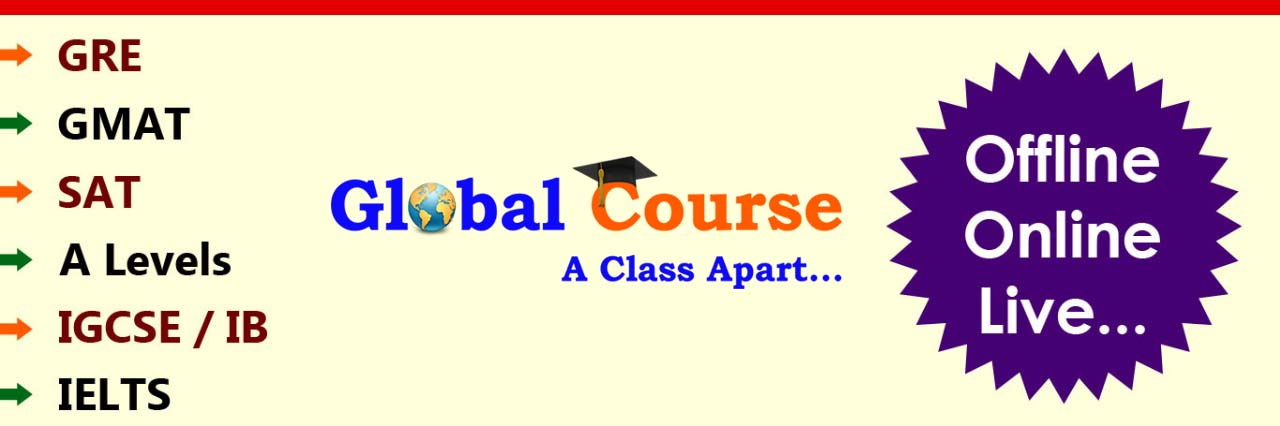•••••NPAT-SYLLABUS - NON ENGINEERING

 Section 1 : Proficiency in English Language Construct Sub construct Error Recognition Recognising grammatical structure and usage. Applied Grammar Using prepositions, determiners, connectives, tenses appropriately. Vocabulary Grasping the meaning of underlined words in sentences Contextual Usage Using appropriate words in the given context Sequencing of Ideas Putting ideas into logical sequence by putting jumbled sentences in the correct order Reading Comprehension (3 in Passages of 400-500 words with 5 Questions per Passage) Locating Information, grasping ideas, identifying relationships, interpreting ideas, moods, characteristics of characters, tone of passage, inferring, getting the central theme, evaluating

 Section 2: Quantitative and Numerical Ability Construct Sub construct Number System Fractions, Surds and Decimals, Number Series Arithmetic Percentages, Profit & Loss, Discount, Compound Interest & Annuities, Ratio & Proportions, Time, Work & Distance, 2 D & 3D Figures- Areas & Volumes Algebra Basic Algebraic Identities, Equations - Linear & Quadratic sequence and Series (AP, GP) Sets and Functions Sets, Operation on Sets and their Applications, using Venn Diagrams, functions Elementary Statistics & Probability Mean, Mode, and Median, Measures of Dispersion Trigonometry Trigonometric Rations, identities, Height and Distances

 Section 3: Reasoning & General Intelligence Construct Sub construct Critical Thinking Decision Making (Take into cognizance various rules/ conditions and take decisions based upon those rules / conditions) Problem Solving (To analyse the given information and condense all the information in a suitable form and answer the questions asked) Verbal-logical reasoning Derive conclusions from logical premises or assess the validity of arguments based on statement of facts Data sufficiency Judge if the information given is sufficient to answer the question or some additional information is required Numerical Reasoning Venn Diagram (Identify the class-sub class relationship among given group of items and illustrate it diagrammatically) Mathematical Equalities Data Interpretation Be able to use the information given in graphs and charts to answer questions Spatial Reasoning Figure Analogy (Choosing the figure from the alternatives that match with relationship specified by a given figural pair) Figure Matching / Classification (Notice the common quality in figures to be able to match figures or find the odd one out) Figure Series (To discover a pattern in the formation of figures in a sequence to be able to complete the series / identify the missing figures)Please fix an appointment before you come to meet...

Malad West : B-302, Rajendra Vihar CHSL,
Next to State Bank Of India, Evershine Nagar,